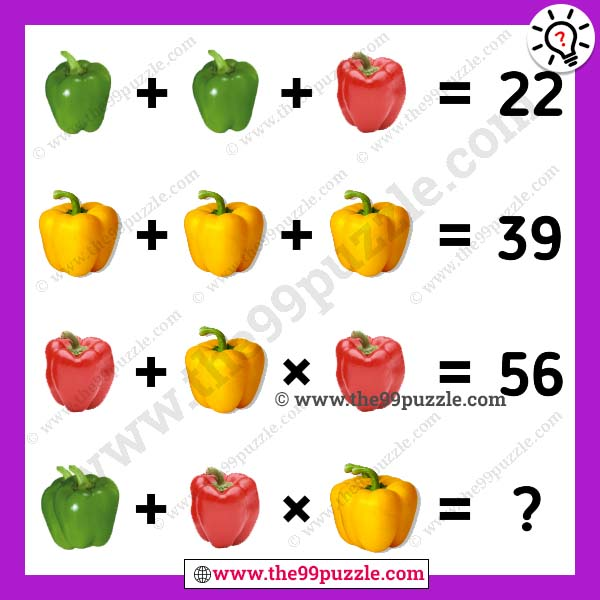# Capsicum puzzle picture tricky equation with answer – Puzz283

Online puzzles help to improve your logical skills. Most puzzlers fail to solve this brain teaser puzzle. In this capsicum puzzle picture tricky equation, you see three different colors of capsicum. Can you solve the last equation? This math picture is simple and more tricky than other picture puzzles. If you practice more math equation puzzles, find this website and try to solve all these puzzles. To learn and solve quickly math tricks by the practice of these puzzles math. If you are smart at puzzles you can solve this problem.###### Explanation:

Green Capsicum = 9

Red Capsicum = 4

Yellow Capsicum = 13

9+9+4 = 22

13+13+13 = 39

4+13×4 = 56

(9+9)+4×(13+13) = 122